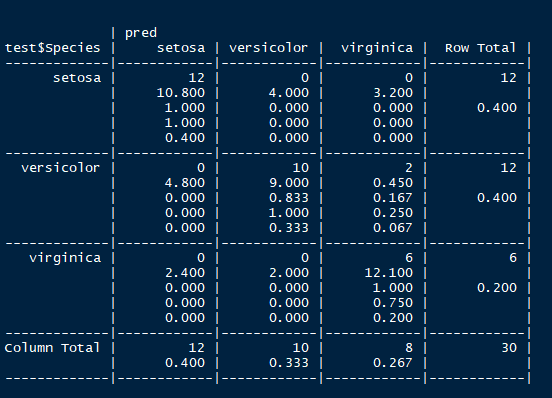# 分类算法1：朴素贝叶斯法及R语言实现

（1）贝叶斯定理
（2）特征条件独立的假设

$P(B_i|A)=\frac{P(A|B_i)P(B_i)}{\sum_{j=1}^{n}P(A|B_j)P(B_ij)}$

$P(B_i|A)=P(A|B_i)P(B_i)$，这也称为贝叶斯定理的核。下面，我们来引入特征条件独立的假设，特征条件独立假设就是假设数据$A$所携带$k$个特征之间是相互独立的，于是，又可以进一步简化，$P(A|B_i)P(B_i)=P(A_1|B_i)P(A_2|B_i)\cdot \cdot \cdot P(A_k|B_i)P(B_i)$，所以就有：$P(B_i|A)=P(A_1|B_i)P(A_2|B_i)\cdot \cdot \cdot P(A_k|B_i)P(B_i)$。这里，我们假设有$q$个类别，朴素贝叶斯法就是求出$P(B_1|A)，P(B_2|A),\cdot \cdot \cdot ,P(B_q|A)$$q$个概率，然后找出最大的哪一个概率，进而进行分类。

## iris data set

# 该数据集有3个类别：setosa & versicolor & virginica
# 4个特征：Sepal.Length & Sepal.Width & Petal.Length & Petal.Width

##-----------------------------------------------------------------------------------

# 详细了解iris数据集

#埃德加安德森的虹膜数据

#虹膜数据集分别给出了3种虹膜中50种花的变量萼片长度和宽度以及花瓣长度和宽度的厘米尺寸。
#物种是鸢尾（Iris setosa），云芝（versicolor）和维吉尼亚（virginica）



step1:构建训练集 & 测试集



##-----------------------------------------------------------------------------------

##  总共150个样本，选120个做为训练集（每个类别选40个样本），剩下的30个作为测试集

### 用抽样的方式构造训练集会更为科学

train_ord <- sample(nrow(iris),120,replace = FALSE)
train <- iris[train_ord,]
test <- iris[-train_ord,]



step2：构建朴素贝叶斯分类器（具体请查看help文档）

library(e1071)
m <- naiveBayes(Species ~., data = train)
pred <- predict(m,test)
library(gmodels)
CrossTable(test\$Species,pred)$X_1,...X_n$是来自总体$X$的样本，$x_1,...,x_n$是它的观察值，那么我们就可以得到$X_1,...X_n$的联合密度：$f(x,\theta)=f(x_1,...,x_n,\theta)$$\theta \in \Theta$是总体X中的参数,$\Theta$是参数空间，显然，从总体中抽样得到的样本信息都包含在了联合密度$f(x,\theta)=f(x_1,...,x_n,\theta)$中，没办法，就是这么强大。经典统计学认为未知参数$\theta$是常数，而贝叶斯统计认为未知参数$\theta$是一个随机变量，这样，样本$X_1,...X_n$的联合密度函数就是在给定$\theta$的条件下的条件密度函数，称为似然函数，即$L(x| \theta)=f(x_1,...,x_n,\theta)$，由于$\theta$是随机变量，故它也具有自己的分布，我们称之为先验分布，这里，用$\pi(\theta)$来表示，一般是由参数的先验信息来确定的，这个地方，就有点神秘了，这里重点理解贝叶斯定理，对如何确定$\pi(\theta)$不做过多的介绍。

$\pi(\theta|x)=\frac{L(x| \theta)\pi(\theta)}{\int_{\Theta}L(x| \theta)\pi(\theta)}$，称为后验密度函数。

 贝叶斯统计学及其应用，韩明，同济大学出版社，2015.6.